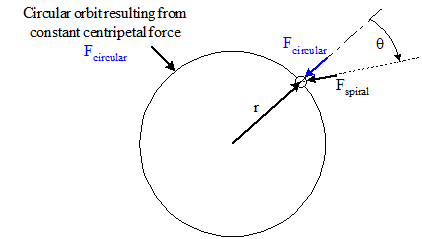# Dynamics of a spiral movement

• irdeg
In summary, a spiral movement is created when a centripetal force increases linearly with time. It can be created with a magnetic field or charged particle moving perpendicularly to the field.

## Homework Statement

As you'll know, the driving force for a circular movement is a centripetal force. But wat is the dynamics for a spiral movement, i.e. an increasing circular movement? And how can I produce it, let's say for a little spherical object, in real life?

## The Attempt at a Solution

I guess there will also be a force parallel to the velocity. I don't know how to create a force that is allways parallel to the velocity.

Hi irdeg,

Welcome to Physics Forums!

Maybe try thinking about what would happen if the "centripetal" force happened to not actually point towards the center, but instead was directed at some constant angle to the radius vector.#### Attachments

irdeg said:
a spiral movement,
Which type of spiral? Geometric, arithmetic...?

gneill said:
Hi irdeg,

Welcome to Physics Forums!

Maybe try thinking about what would happen if the "centripetal" force happened to not actually point towards the center, but instead was directed at some constant angle to the radius vector.

View attachment 232086
So, there will be a component parallel to the velocity and the radius of the circle would grow over time. How could I, practically create something like that?

haruspex said:
Which type of spiral? Geometric, arithmetic...?
I would prefer arithmetic.

Regardless of how an object is moving, its acceleration (and hence the force acting on it) will be perpendicular to the velocity unless the speed is increasing or decreasing. This follows directly from differentiating ##v^2 = \vec v^2## with respect to time.

Now, this means that in an accelerated circular motion (i.e., increasing speed) there is also a force component parallel to the velocity, but this is true regardless of the shape of the motion.

irdeg said:
So, there will be a component parallel to the velocity and the radius of the circle would grow over time. How could I, practically create something like that?
That's a very vague question. What are the boundaries on your definition of "practical"?

•gneill
gneill said:
So a "practical" demonstration might be to have a charged particle moving perpendicularly to an increasing or decreasing magnetic field, hence continuously changing the centripetal force.
Yes, and you would not need it to vary over time. It could be a field which is strongest at some central axis then falling off at greater radii. Depending on the injection parameters the particle could spiral in or out.

••